# Three Dimensional Geometry Class 12 Notes Mathematics

Three Dimensional Geometry Class 12 Notes Mathematics in PDF are available for free download in myCBSEguide mobile app. The best app for CBSE students now provides Three Dimensional Geometry class 12 Notes latest chapter wise notes for quick preparation of CBSE board exams and school-based annual examinations. Class 12 Mathematics notes on chapter 11 Three Dimensional Geometry are also available for download in CBSE Guide website.

## Three Dimensional Geometry Class 12 Notes Mathematics

Download CBSE class 12th revision notes for chapter 11 Three Dimensional Geometry in PDF format for free. Download revision notes for Three Dimensional Geometry class 12 Notes and score high in exams. These are the Three Dimensional Geometry class 12 Notes prepared by team of expert teachers. The revision notes help you revise the whole chapter 11 in minutes. Revision notes in exam days is one of the best tips recommended by teachers during exam days.

## CBSE Class 12 Mathematics Revision Notes Chapter 11 Three Dimensional Geometry

• Direction cosines of a line: Direction cosines of a line are the cosines of the angles made by the line with the positive directions of the coordinate axes.
•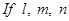are the direct ion cosines of a line, then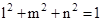• Direct ion cosines of a line joining two points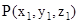and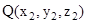are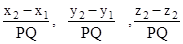·   where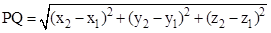• Direction ratios of a line are the numbers which are proportional to the direct ion cosines of a line.
• If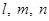are the direct ion cosines and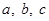are the direct ion ratios of a line

Then,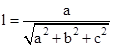,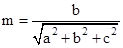,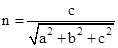• Skew lines: Skew lines are lines in space which are neither parallel nor intersecting. They lie in different planes.
• Angle between two skew lines: Angle between skew lines is the angle between two intersecting lines drawn from any point (preferably through the origin) parallel to each of the skew lines.
• If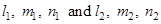are the direction cosines of two lines; and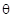is the acute angle between the two lines; then,

• Vector equation of a line that passes through the given point whose position vector is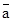and parallel to a given vector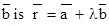• Equation of a line through a point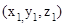and having direct ion cosinesis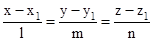• The vector equation of a line which passes through two points whose position vectors are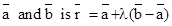• Cartesian equation of a line that passes through two pointsand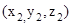is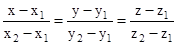• Ifis the acute angle between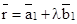and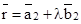then,
• If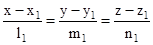and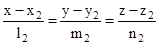are the equations of two lines, then the acute angle between the two lines is given by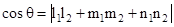• Shortest distance between two skew lines is the line segment perpendicular to both the lines.
• Shortest distance between  and  is
• Shortest distance between the lines: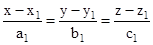and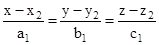is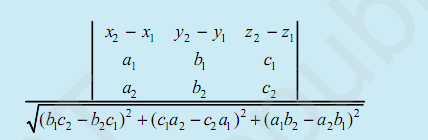• Distance between parallel lines   and is
• In the vector form, equation of a plane which is at a distance d from the origin, and is the unit vector normal to the plane through the origin is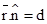• Equation of a plane which is at a distance of d from the origin and the direction cosines of the normal to the plane as l, m, n is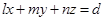.
• The equation of a plane through a point whose position vector is a and perpendicular to the vector  is .
• Equation of a plane perpendicular to a given line with direction ratios A, B, C and passing through a given pointis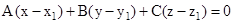• Equation of a plane passing through three non collinear points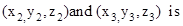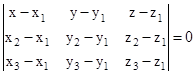• Vector equation of a plane that contains three non collinear points having position vectors and  is  .
• Equation of a plane that cuts the coordinates axes at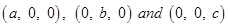is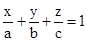.
• Vector equation of a plane that passes through the intersection of planes  and  is ,  where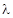is any non-zero constant.
• Cartesian equation of a plane that passes that passes through the intersection of two given planes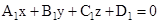and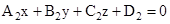is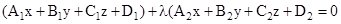• Two lines  and  are coplanar if
• Two planes  and    are coplanar if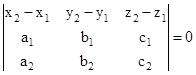• In the vector form, ifis the angle between the two planes,  and , then
• The angle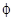between the line  and the plane  is
• The anglebetween the planesandis given by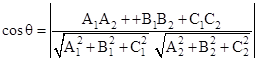• The distance of a point whose position vector is  from the plane   is .
• The distance from a point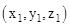to the plane Ax + By + Cz + D = 0 is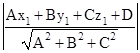• Equation of any plane that is parallel to a plane that is parallel to a plane Ax + By + Cz + D = 0 is Ax + By + Cz + k = 0, where k is a different constant other than D.

## CBSE Class 12 Revision Notes and Key Points

Three Dimensional Geometry class 12 Notes Mathematics. CBSE quick revision note for class-12 Chemistry Physics Maths, Biology and other subject are very helpful to revise the whole syllabus during exam days. The revision notes covers all important formulas and concepts given in the chapter. Even if you wish to have an overview of a chapter, quick revision notes are here to do if for you. These notes will certainly save your time during stressful exam days.

To download Three Dimensional Geometry class 12 Notes Mathematics, sample paper for class 12 Physics, Chemistry, Biology, History, Political Science, Economics, Geography, Computer Science, Home Science, Accountancy, Business Studies, and Home Science; do check myCBSEguide app or website. myCBSEguide provides sample papers with solution, test papers for chapter-wise practice, NCERT Three Dimensional Geometry, NCERT Exemplar Three Dimensional Geometry, quick revision notes for ready reference, CBSE guess papers and CBSE important question papers. Sample Paper all are made available through the best app for CBSE students and myCBSEguide website.### Test Generator

Create question paper PDF and online tests with your own name & logo in minutes.### myCBSEguide

Question Bank, Mock Tests, Exam Papers, NCERT Solutions, Sample Papers, Notes

1. Good